# Fields 2009 Finite Type Invariants Proposal

(diff) ← Older revision | Latest revision (diff) | Newer revision → (diff)

This is a part of a proposal for a 2009 Knot Theory Program at the Fields Institute.

## What is a Universal Finite Type Invariant?

Given a graph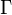$\Gamma$ ("the skeleton"), let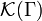${\mathcal K}(\Gamma)$ denote the set of all "knottings" of$\Gamma$ - the set of all embeddings of$\Gamma$ into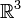${\mathbb R}^3$ considered modulo isotopy. So if$\Gamma$ is a circle,${\mathcal K}(\Gamma)$ is an ordinary knot. If it is a union of circles,${\mathcal K}(\Gamma)$ is a link, and if it is, say, a tetrahedron,${\mathcal K}(\Gamma)$ will contain, for example, the knotted graph shown on the right.

A universal finite type invariant (using a rather broad definition) is a family of invariants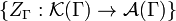$\{Z_\Gamma:{\mathcal K}(\Gamma)\to{\mathcal A}(\Gamma)\}$, one for each skeleton$\Gamma$, valued in some specific family of linear spaces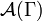${\mathcal A}(\Gamma)$ one for each$\Gamma$. The spaces${\mathcal A}(\Gamma)$ are themselves defined in terms of graphs along with some linear algebra, but since we don't need the details here, we won't show them. A certain "universality" property is expected to hold, but again, we don't need it right now so we won't discuss it.